# Resampling

• 1371 Accesses

## Abstract

Generally, there is not only one statistical model that explains a phenomenon. In that case, the more complicated the model, the easier it is for the statistical model to fit the data. However, we do not know whether the estimation result shows a satisfactory (prediction) performance for new data different from those used for the estimation. For example, in the forecasting of stock prices, even if the price movements up to yesterday are analyzed so that the error fluctuations are reduced, the analysis is not meaningful if no suggestion about stock price movements for tomorrow is given. In this book, choosing a more complex model than a true statistical model is referred to as overfitting. The term overfitting is commonly used in data science and machine learning. However, the definition may differ depending on the situation, so the author felt that uniformity was necessary. In this chapter, we will first learn about cross-validation, a method of evaluating learning performance without being affected by overfitting. Furthermore, the data used for learning are randomly selected, and even if the data follow the same distribution, the learning result may be significantly different. In some cases, the confidence and the variance of the estimated value can be evaluated, as in the case of linear regression. In this chapter, we will continue to learn how to assess the dispersion of learning results, called bootstrapping.

This is a preview of subscription content, access via your institution.

Chapter
USD   29.95
Price excludes VAT (USA)
• DOI: 10.1007/978-981-15-7877-9_4
• Chapter length: 18 pages
• Own it forever
• Exclusive offer for individuals only
• Tax calculation will be finalised during checkout
eBook
USD   34.99
Price excludes VAT (USA)
• ISBN: 978-981-15-7877-9
• Own it forever
• Exclusive offer for individuals only
• Tax calculation will be finalised during checkout
Softcover Book
USD   44.99
Price excludes VAT (USA)
1. 1.

Many books mention a restrictive formula valid only for LOOCV (k = N). This book addresses the general formula applicable to any k.

2. 2.

Linear Model Selection by Cross-Validation Jun Shao, Journal of the American Statistical Association Vol. 88, No. 422 (Jun., 1993), pp. 486–494.

3. 3.

In a portfolio, for two brands X and Y , the quantity of X and Y  is often estimated.

Authors

## Appendices

### Proposition 15 (Sherman–Morrison–Woodbury)

For m, n ≥ 1 and a matrix $$A\in {\mathbb R}^{n\times n},\ U\in {\mathbb R}^{n\times m},\ C\in {\mathbb R}^{m\times m},\ V\in {\mathbb R}^{m\times n}$$ , we have

\displaystyle \begin{aligned} (A+UCV)^{-1}=A^{-1}-A^{-1}U(C^{-1}+VA^{-1}U)^{-1}VA^{-1} \end{aligned}
(4.5)

Proof

The derivation is due to the following:

\displaystyle \begin{aligned} \begin{array}{rcl} & &\displaystyle (A+UCV)(A^{-1}-A^{-1}U(C^{-1}+VA^{-1}U)^{-1}VA^{-1})\\ & &\displaystyle \quad =I+UCVA^{-1}-U(C^{-1}+VA^{-1}U)^{-1}VA^{-1}\\& &\displaystyle \quad \quad -UCVA^{-1}U(C^{-1}+VA^{-1}U)^{-1}VA^{-1}\\ & &\displaystyle \quad =I+UCVA^{-1}-UC\cdot (C^{-1})\cdot (C^{-1}+VA^{-1}U)^{-1}VA^{-1}\\ & &\displaystyle \quad \quad -UC\cdot VA^{-1}U\cdot (C^{-1}+VA^{-1}U)^{-1}VA^{-1}\\ & =&\displaystyle I+UCVA^{-1}- UC(C^{-1}+VA^{-1}U)(C^{-1}+VA^{-1}U)^{-1}VA^{-1}=I. \end{array} \end{aligned}

### Proposition 16

Suppose that X T X is a nonsingular matrix. For each S ⊂{1, …, N}, if $$X_{-S}^TX_{-S}$$ is a nonsingular matrix, so is I  H S.

Proof

For m, n ≥ 1, $$U\in {\mathbb R}^{m\times n}$$, and $$V\in {\mathbb R}^{n\times m}$$, we have

\displaystyle \begin{aligned} \begin{array}{rcl}\left[ \begin{array}{c@{\quad }c} I& 0\\ V& I \end{array} \right] \left[ \begin{array}{c@{\quad }c} I+UV&\displaystyle U\\ 0& I \end{array} \right] \left[ \begin{array}{c@{\quad }c} I&\displaystyle 0\\ -V& I \end{array} \right] &\displaystyle =&\displaystyle \left[ \begin{array}{c@{\quad }c} I+UV&\displaystyle U\\ V+VUV& VU+I \end{array} \right] \left[ \begin{array}{c@{\quad }c} I&\displaystyle 0\\ -V& I \end{array} \right]\\ & =&\displaystyle \left[ \begin{array}{c@{\quad }c} I&\displaystyle U\\ 0& I+VU \end{array} \right]\ . \end{array} \end{aligned}

Combined with Proposition 2, we have

\displaystyle \begin{aligned} \det(I+UV)=\det(I+VU)\ . \end{aligned}
(4.6)

Therefore, from Proposition 2, we have

\displaystyle \begin{aligned} \begin{array}{rcl} \det(X_{-S}^TX_{-S}) & =&\displaystyle \det(X^TX-X_S^TX_S)\\ & =&\displaystyle \det(X^TX)\det(I-(X^TX)^{-1}X_S^TX_S)\\ & =&\displaystyle \det(X^TX)\det(I-X_S(X^TX)^{-1}X_S^T)\ , \end{array} \end{aligned}

where the last transformation is due to (4.6). Hence, from Proposition 1, if $$X_{-S}^TX_{-S}$$ and X T X are nonsingular, so is I − H S. □

### Exercises 32–39

1. 32.

Let m, n ≥ 1. Show that for matrix $$A\in {\mathbb R}^{n\times n},\ U\in {\mathbb R}^{n\times m},\ C\in {\mathbb R}^{m\times m},\ V\in {\mathbb R}^{m\times n}$$,

\displaystyle \begin{aligned} (A+UCV)^{-1}=A^{-1}-A^{-1}U(C^{-1}+VA^{-1}U)^{-1}VA^{-1} \end{aligned}
(4.7)

(Sherman–Morrison–Woodbury). Hint: Continue the following:

\displaystyle \begin{aligned} \begin{array}{rcl} & &\displaystyle (A+UCV)(A^{-1}-A^{-1}U(C^{-1}+VA^{-1}U)^{-1}VA^{-1})\\ & &\displaystyle \quad =I+UCVA^{-1}-U(C^{-1}+VA^{-1}U)^{-1}VA^{-1}\\ & &\displaystyle \quad \quad -UCVA^{-1}U(C^{-1}+VA^{-1}U)^{-1}VA^{-1}\\ & &\displaystyle \quad =I+UCVA^{-1}-UC\cdot (C^{-1})\cdot (C^{-1}+VA^{-1}U)^{-1}VA^{-1}\\ & &\displaystyle \quad \quad -UC\cdot VA^{-1}U\cdot (C^{-1}+VA^{-1}U)^{-1}VA^{-1}. \end{array} \end{aligned}
2. 33.

Let S be a subset of {1, …, N} and write the matrices $$X \in {\mathbb R}^{(N-r)\times (p+1)}$$ that consist of the rows in S and the rows not in S as $$X_S\in {\mathbb R}^{r\times (p+1)}$$ and $$X_{-S}\in {\mathbb R}^{(N-r)\times (p+1)}$$, respectively, where r is the number of elements in S. Similarly, we divide $$y\in {\mathbb R}^{N}$$ into y S and y S.

1. (a)

Show

\displaystyle \begin{aligned}(X_{-S}^TX_{-S})^{-1}=(X^TX)^{-1}+(X^TX)^{-1}X_{S}^T(I-H_{S})^{-1}X_{S}(X^TX)^{-1}\ ,\end{aligned}

where $$H_{S}:=X_{S}(X^TX)^{-1}X_{S}^T$$ is the matrix that consists of the rows and columns in S of H = X(X T X)−1 X T. Hint: Apply n = p + 1, m = r, A = X T X, C = I, $$U=X_{S}^T$$, V = −X S to (4.3).

2. (b)

For $$e_S:=y_S-\hat {y}_S$$ with $$\hat {y}_S=X_S\hat {\beta }$$, show the equation

\displaystyle \begin{aligned} \begin{array}{rcl} \hat{\beta}_{-S}=\hat{\beta}-(X^TX)^{-1}X_{S}^T(I-H_{S})^{-1}e_{S} \end{array} \end{aligned}

Hint: From $$X^TX=X_S^TX_S+X_{-S}^TX_{-S}$$ and $$X^Ty=X_S^Ty_S+X_{-S}^Ty_{-S}$$,

\displaystyle \begin{aligned} \begin{array}{rcl} \hat{\beta}_{-S}& =&\displaystyle \{(X^TX)^{-1}+(X^TX)^{-1}X_{S}^T(I-H_{S})^{-1}X_{S}(X^TX)^{-1}\}(X^Ty-X_{S}^Ty_{S})\\ & =&\displaystyle \hat{\beta}-(X^TX)^{-1}X_{S}^T(I-H_{S})^{-1}(X_{S}\hat{\beta}-H_{S}y_{S})\\ & =&\displaystyle \hat{\beta}-(X^TX)^{-1}X_{S}^T(I-H_{S})^{-1}\{(I-H_{S})y_{S}-X_{S}\hat{\beta}+H_{S}y_{S}\} . \end{array} \end{aligned}
3. 34.

By showing $$y_{S}-X_{S}\hat {\beta }_{-S}=(I-H_{S})^{-1}e_{S}$$, prove that the squared sum of the groups in CV is ∑ S∥(IH S)−1 e S2, where ∥a2 denotes the squared sum of the elements in $$a\in {\mathbb R}^N$$.

4. 35.

Fill in the blanks below and execute the procedure in Problem 34. Observe that the squared sum obtained by the formula and by the general cross-validation method coincide.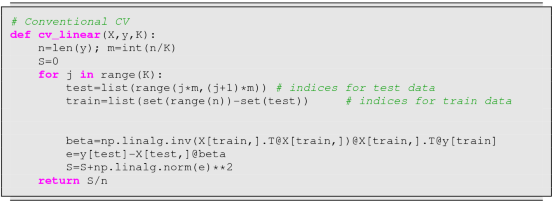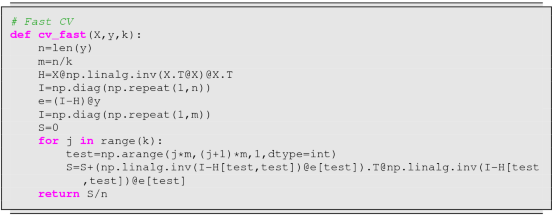Moreover, we wish to compare the speeds of the functions cv_linear and cv_fast. Fill in the blanks below to complete the procedure and draw the graph.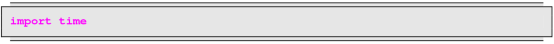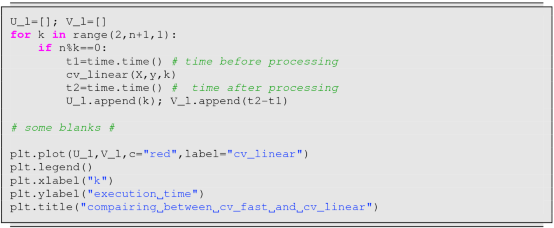Text(0.5, 1.0, 'compairing between cv_fast and cv_linear')

5. 36.

How much the prediction error differs with k in the k-fold CV depends on the data. Fill in the blanks and draw the graph that shows how the CV error changes with k. You may use either the function cv_linear or cv_fast.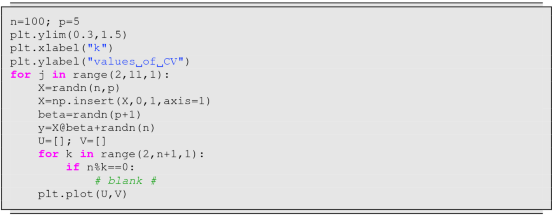6. 37.

We wish to know how the error rate changes with K in the K-nearest neighbor method when 10-fold CV is applied for the Fisher’s Iris data set. Fill in the blanks, execute the procedure, and draw the graph.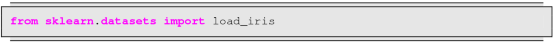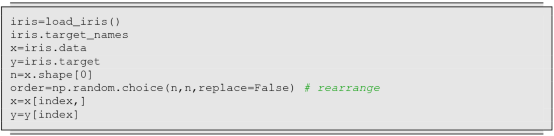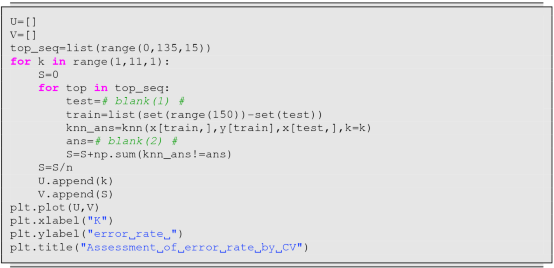Text(0.5, 1.0, 'Assessment of error rate by CV')

7. 38.

We wish to estimate the standard deviation of the quantity below w.r.t. X, Y  based on N data.

\displaystyle \begin{aligned}\frac{v_y-v_x}{v_x+v_y-2v_{xy}} , \ \left\{ \begin{array}{lll} v_x&:=&\displaystyle \frac{1}{N-1}\left[\sum_{i=1}^N X_i^2-\frac{1}{N}\left\{\sum_{i=1}^N X_i\right\}^2\right]\\ {} v_y&:=&\displaystyle \frac{1}{N-1}\left[\sum_{i=1}^N Y_i^2-\frac{1}{N}\left\{\sum_{i=1}^N Y_i\right\}^2\right]\\ {} v_{xy}&:=&\displaystyle \frac{1}{N-1}\left[\sum_{i=1}^N X_iY_i-\frac{1}{N}\left\{\sum_{i=1}^N X_i\right\}\left\{\sum_{i=1}^N Y_i\right\}\right] \end{array} \right. \end{aligned}

To this end, allowing duplication, we randomly choose N data in the data frame r times and estimate the standard deviation (Bootstrap). Fill in the blanks (1)(2) to complete the procedure and observe that it estimates the standard deviation.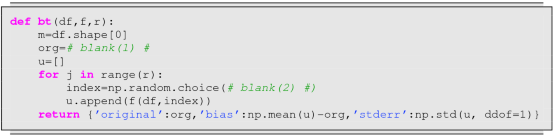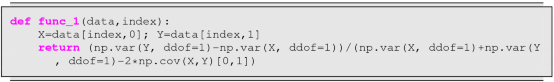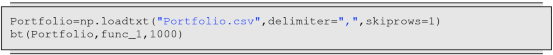8. 39.

For linear regression, if we assume that the noise follows a Gaussian distribution, we can compute the theoretical value of the standard deviation. We wish to compare the value with the one obtained by bootstrap. Fill in the blanks and execute the procedure. What are the three kinds of data that appear first?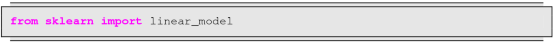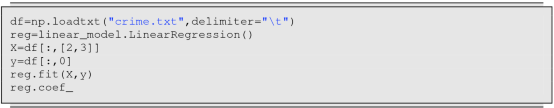array([11.8583308 , -5.97341169])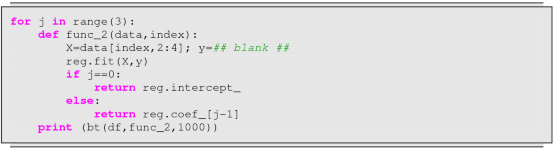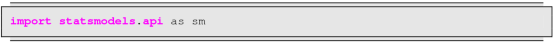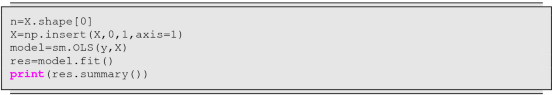## Rights and permissions

Reprints and Permissions